## given 2x – 9y – 12 = 0, express 5x – 4y + 7 = 0 in terms of x only

Question

given 2x – 9y – 12 = 0, express 5x – 4y + 7 = 0 in terms of x only

in progress 0
2 weeks 2021-08-28T18:08:49+00:00 1 Answers 0 views 0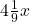+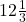= 0

Step-by-step explanation:

Based on 2x – 9y – 12 = 0

-9y = 12 – 2x

9y = -12 + 2x

y =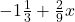Therefore…

5x – 4y + 7 = 0

5x – 4() + 7 = 0

5x +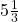–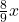+ 7 = 0+= 0